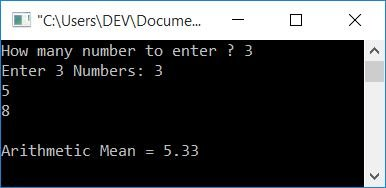# C Program to Calculate Arithmetic Mean

In this article, you will learn and get code about how to find the arithmetic mean of a given list of numbers given by the user (at run-time).

#### How is the Arithmetic Mean calculated?

To calculate the arithmetic mean, use the formula mean = (n1+n2+n2+...+nn)/n. Here, n1 represents the first number, n2 represents the second number, and so on. Whereas n (in the denominator part) is a value that represents the quantity of a number. For example, if there are three numbers, say 3, 5, and 8. So to find its arithmetic mean, use the formula given earlier. Therefore, the arithmetic mean of 3, 5, and 8 will be (3+5+8)/3 or 16/3 or 5.33.

## C Arithmetic Mean Program

To calculate the arithmetic mean in C programming, you have to ask the user to enter how many numbers he or she wants to provide (quantity), say 3. Then ask them to enter all the numbers (say 3, 5, and 8) of that size (3). Now apply the simple formula for finding the arithmetic mean as shown here in the following program:

```#include<stdio.h>
#include<conio.h>
int main()
{
int i, size;
float num, sum, am;
printf("How many number to enter ? ");
scanf("%d", &size);
sum = 0;
printf("Enter %d Numbers: ", size);
for(i=0; i<size; i++)
{
scanf("%f", &num);
sum = sum+num;
}
am = sum/size;
printf("\nArithmetic Mean = %0.2f", am);
getch();
return 0;
}```

This program is compiled and executed using the Code::Blocks IDE. Here is a sample run of the above program:Now supply the size (how many numbers the user wants to enter to find the arithmetic mean of these entered numbers), say 3. Then enter all three numbers, say 3, 5, and 8, and finally press the ENTER key to see the output as shown in the snapshot given below:#### Steps used in previous program

Here is a list of some of the main steps used in the above program:

• Declare any two variables of type int, say size and i.
• Declare another three variables of type float: say num, sum, and am.
• Here, num is used to store the value of each number one by one, sum is used to store the summation of numbers, and am is used to store the arithmetic mean value of all the numbers given by the user.
• Receive the array size from the user.
• Now create a for loop to scan all the numbers of that size. For example, if the user supplies 10 as the size, then create a loop to run 10 times to scan all the 10 numbers one by one.
• After each scan, apply the summation operation, so that after exiting from the loop, you will have a variable named sum that holds the sum of all the numbers given by the user.
• Now just apply the arithmetic mean formula and print the value of am as output.
• The %0.2f format specifier is used to print values up to 2 decimal places only.

#### The same program in different languages

C Quiz

« Previous Program Next Program »